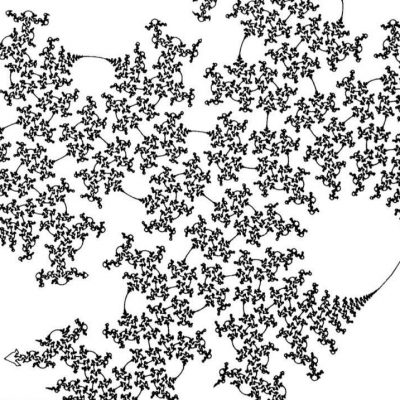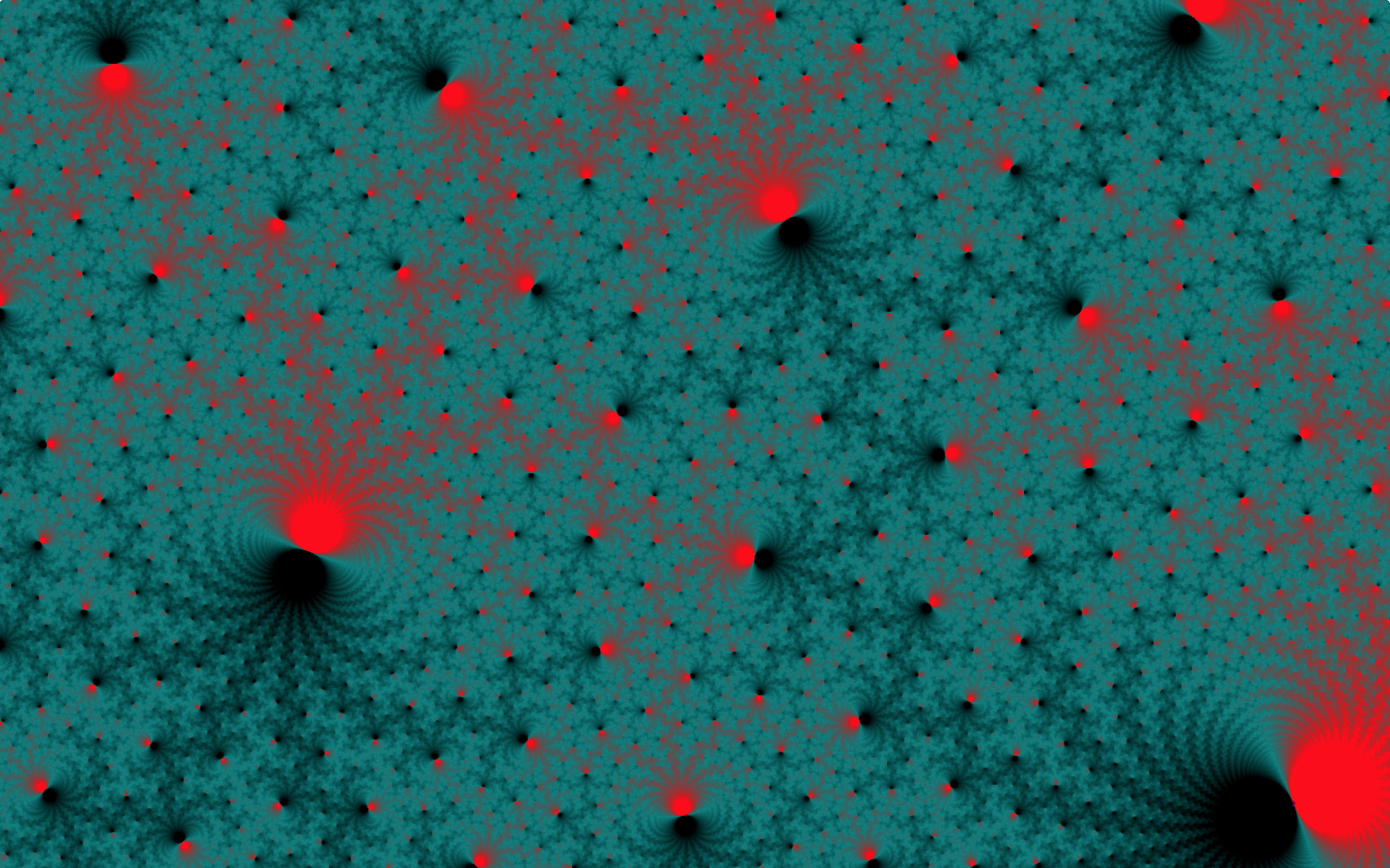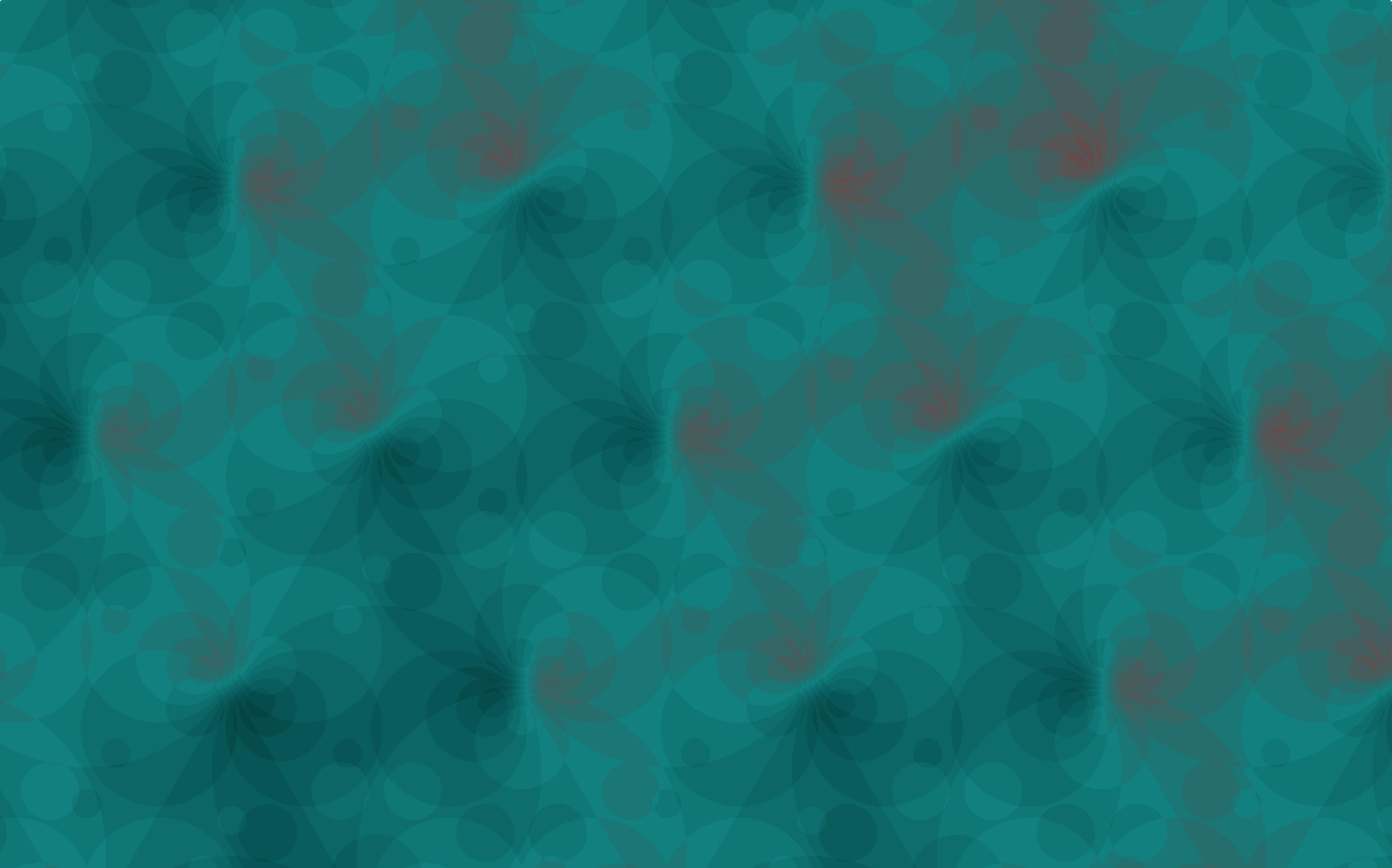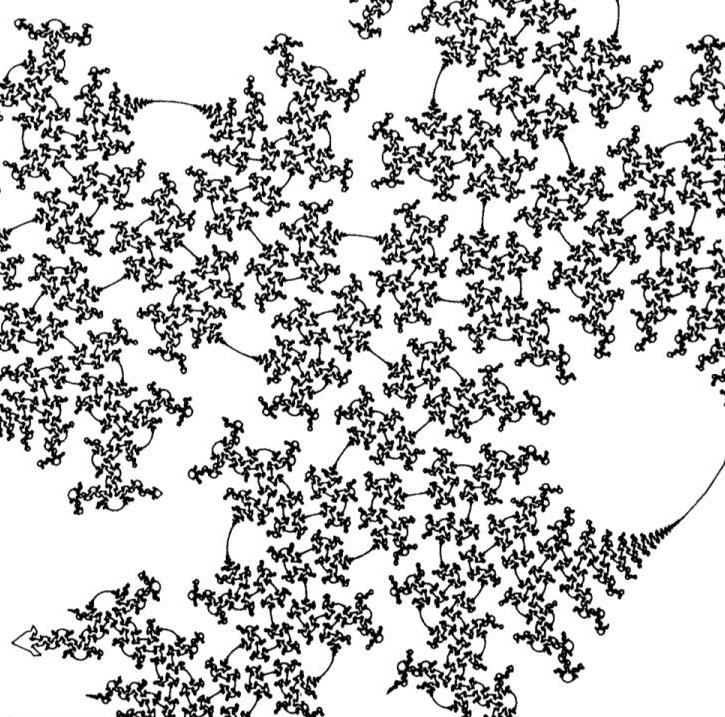# Better pictures of Cannon-Thurston maps

Date started: May 2018
Leads: David Bachman, Saul Schleimer, Henry Segerman

###Abstract

The Cannon-Thurston map is a geometric way to produce sphere-filling curves. Roughly speaking, one starts with a surface$S$ immersed in a three-manifold$M.$ The former has a “circle at infinity”$S^1_\infty$ while the later has a “sphere at infinity”$S^2_\infty$. Thus, sometimes, the immersion$i :S \to M$ leads to a map$i_*$ between the associated objects at infinity. In the good case, when$S$ is a fiber of a fibration of$M$ and when$M$ is hyperbolic, one way to draw the image of$S^1_\infty$ would be to draw a black sphere — after all, the curve is sphere-filling!

Thurston’s classic figure (above right, from this paper) shows an approximation of the sphere-filling curve coming from the inclusion of the Seifert surface$S$ of the figure-eight knot included into its complement$M$. We seek to understand how this picture was drawn, to draw similar pictures for other pairs$(M, S)$, and to find other techniques for drawing Cannon-Thurston maps.

### MediaThe unique taut surface inside of the SnapPy manifold s227. This surface is not a fiber. Thus the image (considered as in the universal cover) is a union of Cannon-Thurston maps for its infinitely many lifts.The Canon-Thurston map for the Seifert surface of the figure-eight knot, as viewed from inside the knot’s complement (m004 in SnapPy notation). A pixel corresponds to a “point near infinity”. The colour of the pixel is computed from the (signed) number of times an interval, from the origin to the point, crosses the surface.A view of a totally geodesic thrice-punctured sphere inside the SnapPy manifold s776. Since the surface is totally geodesic, its lifts to hyperbolic space are all geodesic planes; their boundaries are round circles.The SnapPy manifold s000 is a punctured torus bundle (with monodromy RLLLLL). Here we see the Cannon-Thurston map for the fiber.A screen shot from https://www.shadertoy.com/view/Xd3fzB